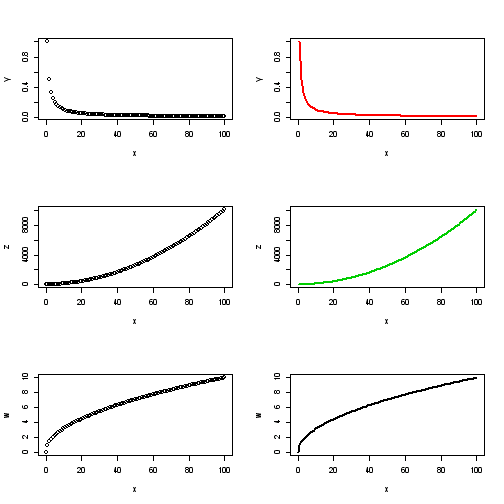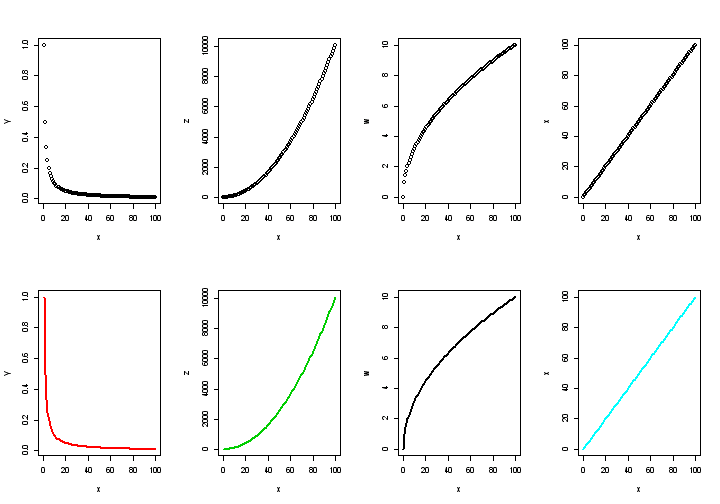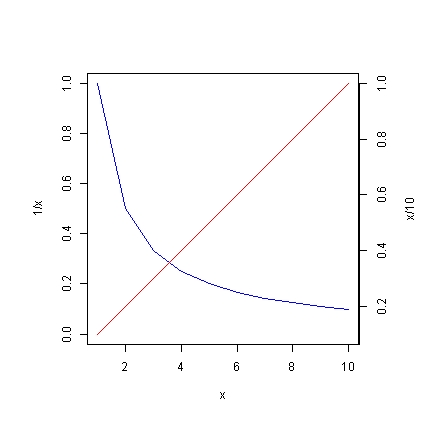## Friday, 6 December 2013

### Simultaneous Display of Multiple Graphs in the same Pane

When you have more than one plots to display together, you can use mfrow() or mfcol() functions to set the layout of the pane to display the graphs. The below example displays 6 graphs in 3 rows and 2 columns using mfcol() function.

x <- c(0:100) y <- 1/x z <- x^2 + x w <- sqrt(x)

par(mfcol = c(3, 2))
plot(x, y)
plot(x, z)
plot(x, w)
plot(x, y, type = "l", lty = 1, lwd = 2, col = 2)
plot(x, z, type = "l", lty = 1, lwd = 2, col = 3)
plot(x, w, type = "l", lty = 1, lwd = 2, col = 9)The below example uses mfrow() function to display 8 graphs in 2 rows and 4 columns.

par(mfrow = c(2, 4)) plot(x, y) plot(x, z) plot(x, w) plot(x, x) plot(x, y, type = "l", lty = 1, lwd = 2, col = 2) plot(x, z, type = "l", lty = 1, lwd = 2, col = 3) plot(x, w, type = "l", lty = 1, lwd = 2, col = 9) plot(x, x, type = "l", lty = 1, lwd = 2, col = 5)### Opening a New Graphic Device

When you are plotting more than one graph in succession, R automatically replace the graphs with the latest one. If you want to retain the older graphs while opening the new ones in separate graphic devices, x11() or windows() is used in Windows OS;  x11() is used in Unix, and quartz() is used in OS X.

x11() plot(1:10) windows() plot(rnorm(10))

### Overlaying an Existing Graph with a New Plot

If you want to overlay a graph with another graph, use par(new=TRUE) between plot() functions as shown in the below example.

x <- 1:10 par(oma = c(1, 2, 1, 3)) plot(1/x ~ x, type = "l", col = "blue", ylim = c(0, 1)) par(new = TRUE) plot(x/10 ~ x, type = "l", col = "red", yaxt = "n", ylab = "") axis(4, at = seq(0, 1, by = 0.2), pos = c(10.4, 0), col = "black", lty = 1) mtext("x/10", 4, line = 3)### Output Graphs into Files

If you want to send the graphic outputs to files instead of displaying them in graphic devices, you can use the below method.

- pdf() will save the output in pdf format;
- jpeg() will save in jpg format;
- png() will save in png format;
- bmp() will save in bmp format, and
- tiff() will save in tif format.

You need to always use dev.off() to deactivate the output device. You also need to ensure that the extension of the assigned file name is consistent with the output device (e.g. file_name.jpg for jpeg() device). Running multiple plot function after an output device is activated before turning it off will save those plots in multiple pages of the assigned file format.

x<-1:100 pdf("file_name.pdf", width=10, height=8) plot(x^2~x,type="l") dev.off()